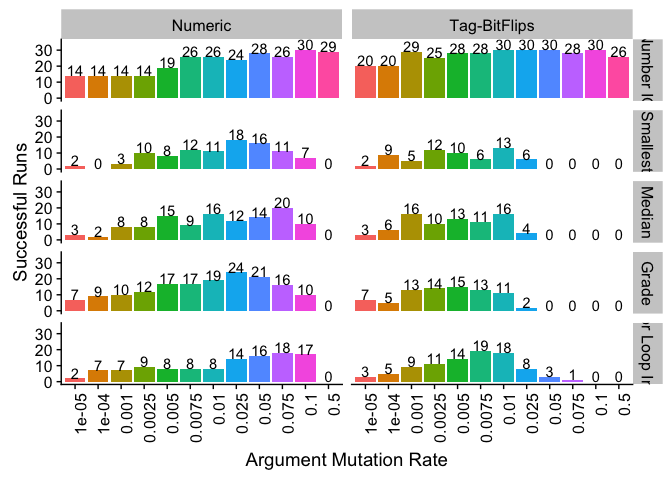## Overview

Here, we analyze our experimental results comparing tag-accessed memory to more traditional, direct-indexed memory. Specifically, we conducted a series of experiments using simple linear-GP representations on program synthesis problems. In all experiments, we evolved genetic programs that used tag-accessed memory and programs that used direct-indexed memory. Aside from how programs were allowed to access memory, both genetic programming systems/representations were identical (e.g., had identical sets of instructions).

First, we present data from our preliminary experiments, which used a range of numeric argument and tag-based argument mutation rates. Based on these preliminary data, we decided to remove our two most extreme mutation rates and run a higher-replicate-count experiment with new random number seeds.

This document was generated using R markdown with R version 3.3.2 (2016-10-31) (R Core Team, 2016).

## Package Setup

``````library(tidyr)    # (Wickham & Henry, 2018)
library(ggplot2)  # (Wickham, 2009)
library(plyr)     # (Wickham, 2011)
library(dplyr)    # (Wickham et al., 2018)
library(cowplot)  # (Wilke, 2018)``````

Set path information (for both preliminary data and for final experiment data).

``````prelim_u300_summary_loc <-
"../data/prelim/min_programs__update_300__solutions_summary.csv"

u500_summary_loc <-
"../data/sweep/min_programs__update_500__solutions_summary.csv"``````

Load data in from file(s). Pretty it up (for our graphs).

``````# Load preliminary data.
prelim_u300_summary <- read.csv(prelim_u300_summary_loc, na.strings = "NONE")
prelim_u300_summary\$arg_mut_rate <- as.factor(prelim_u300_summary\$arg_mut_rate)

prelim_u300_summary\$problem <- factor(prelim_u300_summary\$problem,
levels = c("number-io", "smallest", "median", "grade", "for-loop-index"))
levels(prelim_u300_summary\$problem) <- c("Number IO", "Smallest",

u500_summary <- read.csv(u500_summary_loc, na.strings = "NONE")
u500_summary\$arg_mut_rate <- as.factor(u500_summary\$arg_mut_rate)

u500_summary\$problem <- factor(u500_summary\$problem, levels = c("number-io",
levels(u500_summary\$problem) <- c("Number IO", "Smallest", "Median",

## Preliminary Results

We ran a set of preliminary experiments, applying our simple linear GP representation (with tag-based memory and direct-indexed memory) to 5 problems from the general program synthesis benchmark suite (Helmuth & Spector, 2015): for loop index, grade, median, small or large, and smallest.

We tried several tag-argument and numeric-argument mutation rates in our preliminary runs. For runs that used tag-based arguments, we tried the following per-bit tag-argument mutation rates: 0.00001, 0.0001, 0.001, 0.0025, 0.005, 0.0075, 0.01, 0.025, 0.05, 0.075, 0.1, 0.5. For runs that used numeric arguments, we tried the followign per-argument mutation rates: 0.00001, 0.0001, 0.001, 0.0025, 0.005, 0.0075, 0.01, 0.025, 0.05, 0.075, 0.1, 0.5.

We ran the number io problem for 100 generations and 300 generations for all other problems. For each problem, we looked at the proportion of runs (30 replicates per condition) that produced solutions.

### Successful Runs after 300 Generations

``## Saving 7 x 5 in image``## Experimental Results

In our second (final) set of runs, we applied our two linear GP representations (one with tag-accessed memory and one with direct-indexed memory) to five problems: number IO, for loop index, grade, median, and smallest.

We ran number IO for 100 generations. To increase the solve rates of the other problems, we increased the number of generations we ran for loop index, grade, median, and smallest runs from 300 to 500 generations.

Because the most extreme mutation rates (0.00001 and 0.5) were never the best mutation rate for finding solutions, we dropped them from this experiment to dedicate more computational resources toward running more replicates.

As before, for each problem we looked at the proportion of runs (50 replicates per condition) that produced solutions.

### Successful Runs after 500 Generations

``## Saving 7 x 5 in image``